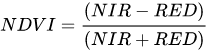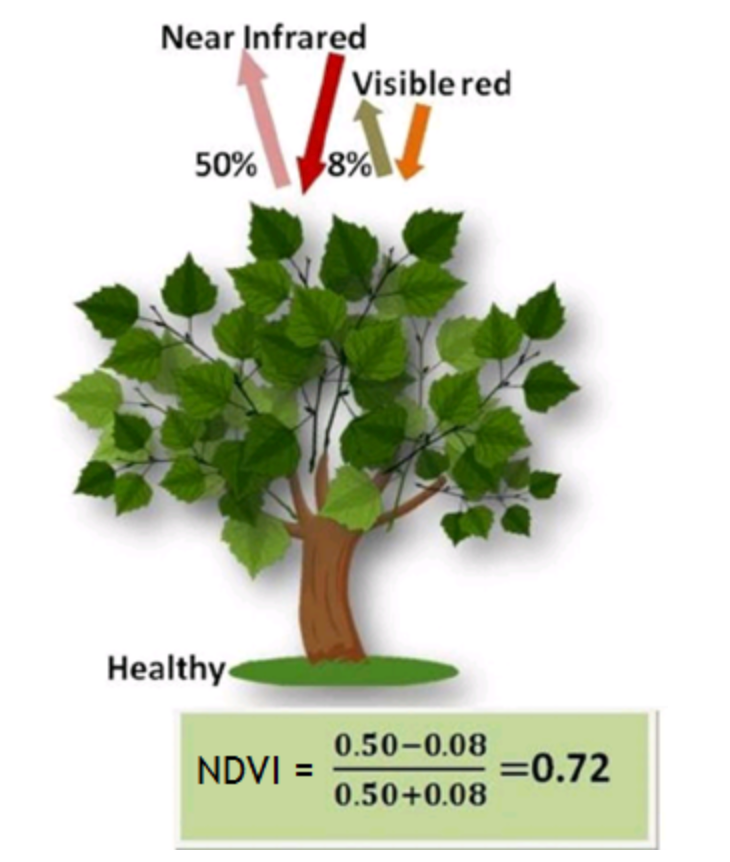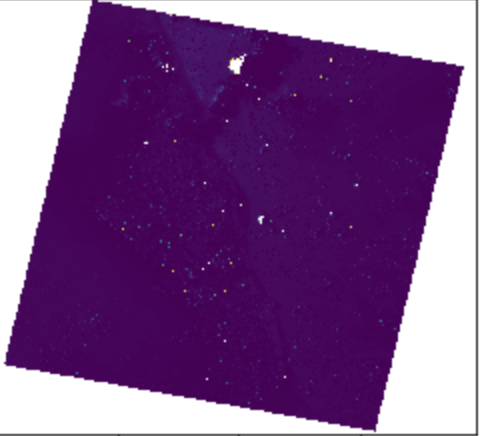#2019 iT 邦幫忙鐵人賽DAY 27
1
AI & Data

## Day27 計算NDVI

• NDVI
• NDVI計算: rasterio, numpy

## NDVI

NDVI是常態化植生指標參考wiki常態化差值植生指標，計算方式是利用紅光與近紅外光波段，代表植物生長與覆蓋的指數，其數值愈大表示植物生長愈多。NIR為近紅外光反射；RED為紅光反射，NDVI之值介於-1到1之間。 當RED=0時，有最大值1；反之，當NIR=0時，有最小值-1。
(取自wiki常態化差值植生指標Calculate NDVI & Extract Spectra Using Masks in Python - Flightline Data | NSF NEON | Open Data to Understand our Ecosystems)

## NDVI計算: rasterio, numpy

``````import rasterio
src=rasterio.open('data/rasterio/data/test.tif')

``````

``````b1, b2, b3, b4 = src.read()
``````

``````import numpy
ndvi = numpy.zeros(b1.shape)
ndvi = (b1-b2)/(b1+b2)
``````

``````import matplotlib.pyplot as plt
from rasterio import plot
%matplotlib inline
plot.show(ndvi)
``````## 後記

EarthExplorer - Home